# DISP-2003_L01B_20Feb03.ppt

16 de Jul de 2022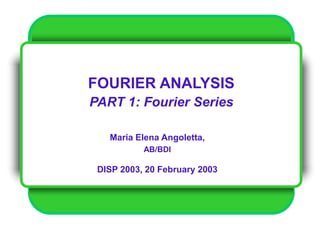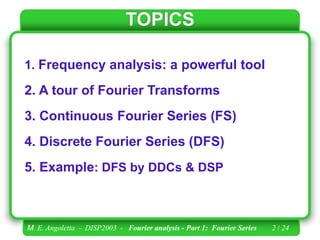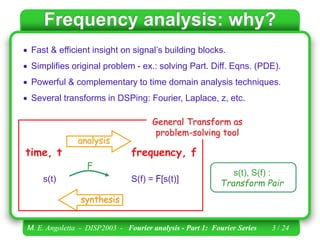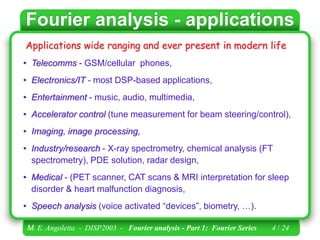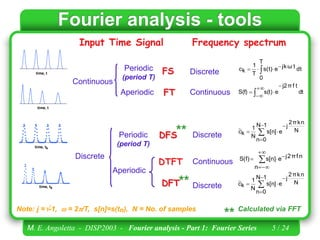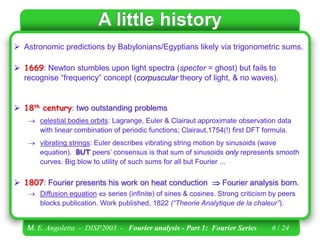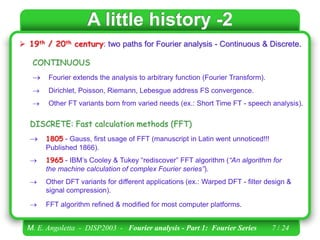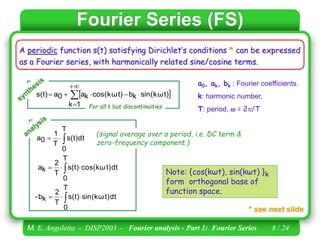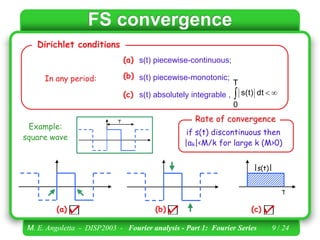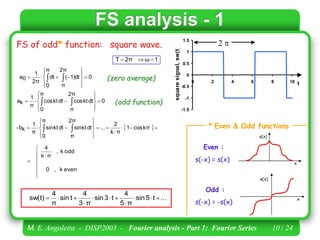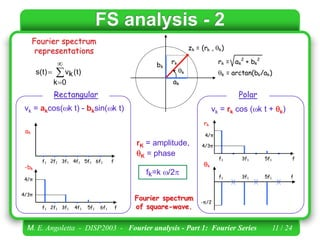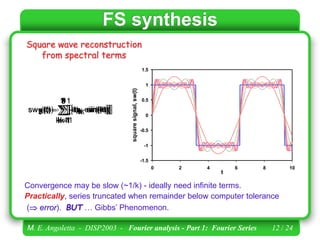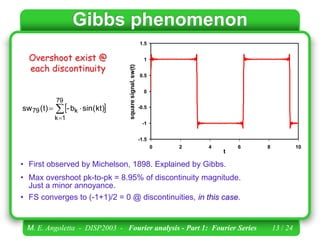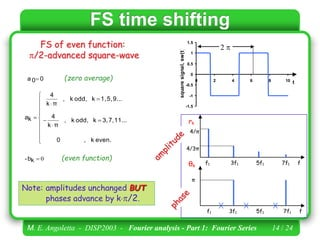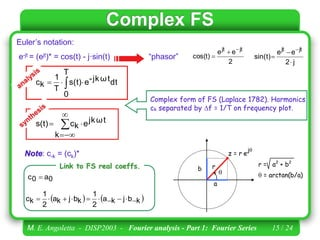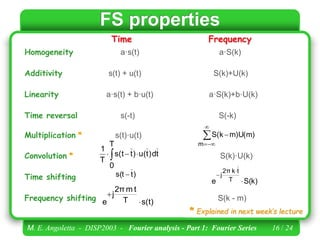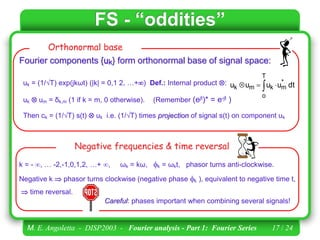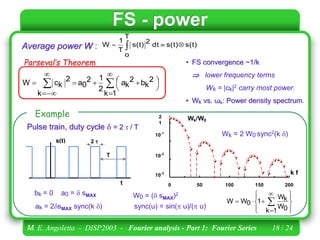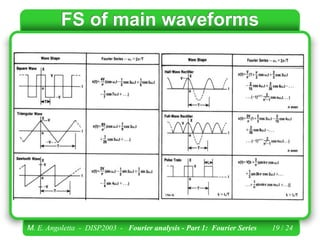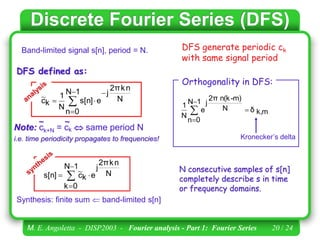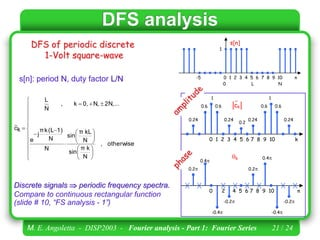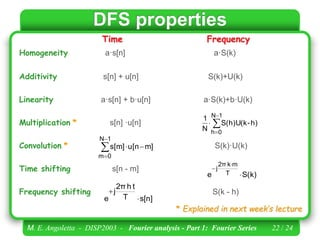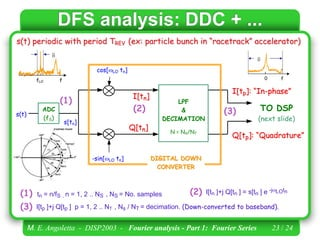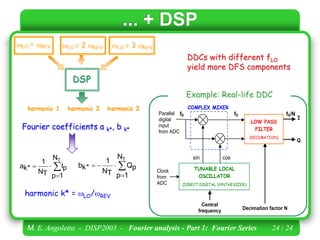1 de 24

### DISP-2003_L01B_20Feb03.ppt

• 1. FOURIER ANALYSIS PART 1: Fourier Series Maria Elena Angoletta, AB/BDI DISP 2003, 20 February 2003
• 2. M. E. Angoletta - DISP2003 - Fourier analysis - Part 1: Fourier Series 2 / 24 TOPICS 1. Frequency analysis: a powerful tool 2. A tour of Fourier Transforms 3. Continuous Fourier Series (FS) 4. Discrete Fourier Series (DFS) 5. Example: DFS by DDCs & DSP
• 3. M. E. Angoletta - DISP2003 - Fourier analysis - Part 1: Fourier Series 3 / 24 Frequency analysis: why?  Fast & efficient insight on signal’s building blocks.  Simplifies original problem - ex.: solving Part. Diff. Eqns. (PDE).  Powerful & complementary to time domain analysis techniques.  Several transforms in DSPing: Fourier, Laplace, z, etc. time, t frequency, f F s(t) S(f) = F[s(t)] analysis synthesis s(t), S(f) : Transform Pair General Transform as problem-solving tool
• 4. M. E. Angoletta - DISP2003 - Fourier analysis - Part 1: Fourier Series 4 / 24 Fourier analysis - applications Applications wide ranging and ever present in modern life • Telecomms - GSM/cellular phones, • Electronics/IT - most DSP-based applications, • Entertainment - music, audio, multimedia, • Accelerator control (tune measurement for beam steering/control), • Imaging, image processing, • Industry/research - X-ray spectrometry, chemical analysis (FT spectrometry), PDE solution, radar design, • Medical - (PET scanner, CAT scans & MRI interpretation for sleep disorder & heart malfunction diagnosis, • Speech analysis (voice activated “devices”, biometry, …).
• 5. M. E. Angoletta - DISP2003 - Fourier analysis - Part 1: Fourier Series 5 / 24 Fourier analysis - tools Input Time Signal Frequency spectrum       1 N 0 n N n k π 2 j e s[n] N 1 k c ~ Discrete Discrete DFS Periodic (period T) Continuous DTFT Aperiodic Discrete DFT n f π 2 j e n s[n] S(f)         0 0.5 1 1.5 2 2.5 0 2 4 6 8 10 12 time, tk 0 0.5 1 1.5 2 2.5 0 1 2 3 4 5 6 7 8 time, tk       1 N 0 n N n k π 2 j e s[n] N 1 k c ~ ** ** Calculated via FFT ** dt t f π j2 e s(t) S(f)         dt T 0 t ω k j e s(t) T 1 k c      Periodic (period T) Discrete Continuous FT Aperiodic FS Continuous 0 0.5 1 1.5 2 2.5 0 1 2 3 4 5 6 7 8 time, t 0 0.5 1 1.5 2 2.5 0 2 4 6 8 10 12 time, t Note: j =-1,  = 2/T, s[n]=s(tn), N = No. of samples
• 6. M. E. Angoletta - DISP2003 - Fourier analysis - Part 1: Fourier Series 6 / 24 A little history  Astronomic predictions by Babylonians/Egyptians likely via trigonometric sums.  1669: Newton stumbles upon light spectra (specter = ghost) but fails to recognise “frequency” concept (corpuscular theory of light, & no waves).  18th century: two outstanding problems  celestial bodies orbits: Lagrange, Euler & Clairaut approximate observation data with linear combination of periodic functions; Clairaut,1754(!) first DFT formula.  vibrating strings: Euler describes vibrating string motion by sinusoids (wave equation). BUT peers’ consensus is that sum of sinusoids only represents smooth curves. Big blow to utility of such sums for all but Fourier ...  1807: Fourier presents his work on heat conduction  Fourier analysis born.  Diffusion equation  series (infinite) of sines & cosines. Strong criticism by peers blocks publication. Work published, 1822 (“Theorie Analytique de la chaleur”).
• 7. M. E. Angoletta - DISP2003 - Fourier analysis - Part 1: Fourier Series 7 / 24 A little history -2  19th / 20th century: two paths for Fourier analysis - Continuous & Discrete. CONTINUOUS  Fourier extends the analysis to arbitrary function (Fourier Transform).  Dirichlet, Poisson, Riemann, Lebesgue address FS convergence.  Other FT variants born from varied needs (ex.: Short Time FT - speech analysis). DISCRETE: Fast calculation methods (FFT)  1805 - Gauss, first usage of FFT (manuscript in Latin went unnoticed!!! Published 1866).  1965 - IBM’s Cooley & Tukey “rediscover” FFT algorithm (“An algorithm for the machine calculation of complex Fourier series”).  Other DFT variants for different applications (ex.: Warped DFT - filter design & signal compression).  FFT algorithm refined & modified for most computer platforms.
• 8. M. E. Angoletta - DISP2003 - Fourier analysis - Part 1: Fourier Series 8 / 24 Fourier Series (FS) * see next slide A periodic function s(t) satisfying Dirichlet’s conditions * can be expressed as a Fourier series, with harmonically related sine/cosine terms.            1 k t) ω (k sin k b t) ω (k cos k a 0 a s(t) a0, ak, bk : Fourier coefficients. k: harmonic number, T: period,  = 2/T For all t but discontinuities Note: {cos(kωt), sin(kωt) }k form orthogonal base of function space.    T 0 s(t)dt T 1 0 a     T 0 dt t) ω sin(k s(t) T 2 k b -     T 0 dt t) ω cos(k s(t) T 2 k a (signal average over a period, i.e. DC term & zero-frequency component.)
• 9. M. E. Angoletta - DISP2003 - Fourier analysis - Part 1: Fourier Series 9 / 24 FS convergence s(t) piecewise-continuous; s(t) piecewise-monotonic; s(t) absolutely integrable ,    T 0 dt s(t) (a) (b) (c) Dirichlet conditions In any period: Example: square wave T (a) (b) T s(t) (c) if s(t) discontinuous then |ak|<M/k for large k (M>0) Rate of convergence
• 10. M. E. Angoletta - DISP2003 - Fourier analysis - Part 1: Fourier Series 10 / 24 FS analysis - 1 * Even & Odd functions Odd : s(-x) = -s(x) x s(x) s(x) x Even : s(-x) = s(x) FS of odd* function: square wave. -1.5 -1 -0.5 0 0.5 1 1.5 0 2 4 6 8 10 t square signal, sw(t)  0 π 0 2π π 1)dt ( dt 2π 1 0 a                  0 π 0 2π π dt kt cos dt kt cos π 1 k a                                       kπ cos 1 π k 2 ... π 0 2π π dt kt sin dt kt sin π 1 k b -          even k , 0 odd k , π k 4 1 ω 2π T    (zero average) (odd function) ... t 5 sin π 5 4 t 3 sin π 3 4 t sin π 4 sw(t)           
• 11. M. E. Angoletta - DISP2003 - Fourier analysis - Part 1: Fourier Series 11 / 24 FS analysis - 2 -π/2 f1 3f1 5f1 f f1 3f1 5f1 f rk θk 4/π 4/3π fk=k /2 rK = amplitude, K = phase rk k ak bk k = arctan(bk/ak) rk = ak 2 + bk 2 zk = (rk , k) vk = rk cos (k t + k) Polar Fourier spectrum representations     0 k (t) k v s(t) Rectangular vk = akcos(k t) - bksin(k t) 4/π f1 2f1 3f1 4f1 5f1 6f1 f f1 2f1 3f1 4f1 5f1 6f1 f 4/3π ak -bk Fourier spectrum of square-wave.
• 12. M. E. Angoletta - DISP2003 - Fourier analysis - Part 1: Fourier Series 12 / 24       7 1 k sin(kt) k b - (t) 7 sw -1.5 -1 -0.5 0 0.5 1 1.5 0 2 4 6 8 10 t square signal, sw(t) -1.5 -1 -0.5 0 0.5 1 1.5 0 2 4 6 8 10 t square signal, sw(t)       5 1 k sin(kt) k b - (t) 5 sw       3 1 k sin(kt) k b - (t) 3 sw -1.5 -1 -0.5 0 0.5 1 1.5 0 2 4 6 8 10 t square signal, sw(t)       1 1 k sin(kt) k b - (t) 1 sw -1.5 -1 -0.5 0 0.5 1 1.5 0 2 4 6 8 10 t square signal, sw(t) -1.5 -1 -0.5 0 0.5 1 1.5 0 2 4 6 8 10 t square signal, sw(t)       9 1 k sin(kt) k b - (t) 9 sw -1.5 -1 -0.5 0 0.5 1 1.5 0 2 4 6 8 10 t square signal, sw(t) -1.5 -1 -0.5 0 0.5 1 1.5 0 2 4 6 8 10 t square signal, sw(t)       11 1 k sin(kt) k b - (t) 11 sw FS synthesis Square wave reconstruction from spectral terms Convergence may be slow (~1/k) - ideally need infinite terms. Practically, series truncated when remainder below computer tolerance ( error). BUT … Gibbs’ Phenomenon.
• 13. M. E. Angoletta - DISP2003 - Fourier analysis - Part 1: Fourier Series 13 / 24 Gibbs phenomenon -1.5 -1 -0.5 0 0.5 1 1.5 0 2 4 6 8 10 t square signal, sw(t)       79 1 k k 79 sin(kt) b - (t) sw Overshoot exist @ each discontinuity • Max overshoot pk-to-pk = 8.95% of discontinuity magnitude. Just a minor annoyance. • FS converges to (-1+1)/2 = 0 @ discontinuities, in this case. • First observed by Michelson, 1898. Explained by Gibbs.
• 14. M. E. Angoletta - DISP2003 - Fourier analysis - Part 1: Fourier Series 14 / 24 FS time shifting -1.5 -1 -0.5 0 0.5 1 1.5 0 2 4 6 8 10 t square signal, sw(t)  FS of even function: /2-advanced square-wave π f1 3f1 5f1 7f1 f f1 3f1 5f1 7f1 f rk θk 4/π 4/3π 0 0 a  0  k b -                even. k , 0 11... 7, 3, k odd, k , π k 4 9... 5, 1, k odd, k , π k 4 k a (even function) (zero average) Note: amplitudes unchanged BUT phases advance by k/2.
• 15. M. E. Angoletta - DISP2003 - Fourier analysis - Part 1: Fourier Series 15 / 24 Complex FS Complex form of FS (Laplace 1782). Harmonics ck separated by f = 1/T on frequency plot. r  a b  = arctan(b/a) r = a2 + b2 z = r e j 2 e e cos(t) jt jt    j 2 e e sin(t) jt jt     Euler’s notation: e-jt = (ejt)* = cos(t) - j·sin(t) “phasor” Note: c-k = (ck)*     k b j k a 2 1 k b j k a 2 1 k c           0 a 0 c  Link to FS real coeffs.       k t ω k j e k c s(t)     T 0 dt t ω k j - e s(t) T 1 k c
• 16. M. E. Angoletta - DISP2003 - Fourier analysis - Part 1: Fourier Series 16 / 24 FS properties Time Frequency * Explained in next week’s lecture Homogeneity a·s(t) a·S(k) Additivity s(t) + u(t) S(k)+U(k) Linearity a·s(t) + b·u(t) a·S(k)+b·U(k) Time reversal s(-t) S(-k) Multiplication * s(t)·u(t) Convolution * S(k)·U(k) Time shifting Frequency shifting S(k - m)      m m)U(m) S(k t d ) t T 0 u( ) t s(t T 1     S(k) e T t k 2π j    s(t) T t m 2π j e   ) t s(t 
• 17. M. E. Angoletta - DISP2003 - Fourier analysis - Part 1: Fourier Series 17 / 24 FS - “oddities” k = - , … -2,-1,0,1,2, …+ , ωk = kω, k = ωkt, phasor turns anti-clockwise. Negative k  phasor turns clockwise (negative phase k ), equivalent to negative time t,  time reversal. Negative frequencies & time reversal Fourier components {uk} form orthonormal base of signal space: uk = (1/T) exp(jkωt) (|k| = 0,1 2, …+) Def.: Internal product : uk  um = δk,m (1 if k = m, 0 otherwise). (Remember (ejt)* = e-jt ) Then ck = (1/T) s(t)  uk i.e. (1/T) times projection of signal s(t) on component uk Orthonormal base     T o * m k m k dt u u u u Careful: phases important when combining several signals!
• 18. M. E. Angoletta - DISP2003 - Fourier analysis - Part 1: Fourier Series 18 / 24 0 50 100 150 200 k f Wk/W0 10-3 10-2 10-1 1 2 Wk = 2 W0 sync2(k ) W0 = ( sMAX)2                 1 k 0 W k W 1 0 W W FS - power • FS convergence ~1/k  lower frequency terms Wk = |ck|2 carry most power. • Wk vs. ωk: Power density spectrum. Example sync(u) = sin( u)/( u) Pulse train, duty cycle  = 2 t/ T T 2 t t s(t) bk = 0 a0 =  sMAX ak = 2sMAX sync(k ) Average power W : s(t) s(t) T o dt 2 s(t) T 1 W                      1 k 2 k b 2 k a 2 1 2 0 a k 2 k c W Parseval’s Theorem
• 19. M. E. Angoletta - DISP2003 - Fourier analysis - Part 1: Fourier Series 19 / 24 FS of main waveforms
• 20. M. E. Angoletta - DISP2003 - Fourier analysis - Part 1: Fourier Series 20 / 24 Discrete Fourier Series (DFS) N consecutive samples of s[n] completely describe s in time or frequency domains. DFS generate periodic ck with same signal period      1 N 0 k N n k 2π j e k c s[n] ~ Synthesis: finite sum  band-limited s[n] Band-limited signal s[n], period = N. m k, δ 1 N 0 n N -m) n(k 2π j e N 1     Kronecker’s delta Orthogonality in DFS:       1 N 0 n N n k 2π j e s[n] N 1 k c ~ Note: ck+N = ck  same period N i.e. time periodicity propagates to frequencies! DFS defined as: ~ ~
• 21. M. E. Angoletta - DISP2003 - Fourier analysis - Part 1: Fourier Series 21 / 24 DFS analysis DFS of periodic discrete 1-Volt square-wave                               otherwise , N k π sin N kL π sin N N 1) (L k π j e 2N,... N, 0, k , N L k c ~ 0.6 0 1 2 3 4 5 6 7 8 9 10 k 1 0 2 4 5 6 7 8 9 10 n k -0.4 0.2 0.24 0.24 0.6 0.6 0.24 1 0.24 -0.2 0.4 0.2 -0.4 -0.2 0.4 0.2 0.6 ck ~ Discrete signals  periodic frequency spectra. Compare to continuous rectangular function (slide # 10, “FS analysis - 1”) -5 0 1 2 3 4 5 6 7 8 9 10 n 0 L N s[n] 1 s[n]: period N, duty factor L/N
• 22. M. E. Angoletta - DISP2003 - Fourier analysis - Part 1: Fourier Series 22 / 24 DFS properties Time Frequency Homogeneity a·s[n] a·S(k) Additivity s[n] + u[n] S(k)+U(k) Linearity a·s[n] + b·u[n] a·S(k)+b·U(k) Multiplication * s[n] ·u[n] Convolution * S(k)·U(k) Time shifting s[n - m] Frequency shifting S(k - h)     1 N 0 h h) - S(h)U(k N 1 * Explained in next week’s lecture      1 N 0 m m] u[n s[m] S(k) e T m k 2π j    s[n] T t h 2π j e  
• 23. M. E. Angoletta - DISP2003 - Fourier analysis - Part 1: Fourier Series 23 / 24 Q Q[ [t tp p] ]: : “ “Q Qu ua ad dr ra at tu ur re e” ” s[tn] s(t) cos[LO tn] I I[ [t tn n] ] -sin[LO tn] Q Q[ [t tn n] ] ADC (fS) I I[ [t tp p] ]: : “ “I In n- -p ph ha as se e” ” LPF & DECIMATION N = NS/NT TO DSP (next slide) D DI IG GI IT TA AL L D DO OW WN N C CO ON NV VE ER RT TE ER R DFS analysis: DDC + ... s(t) periodic with period TREV (ex: particle bunch in “racetrack” accelerator) tn = n/fS , n = 1, 2 .. NS , NS = No. samples (1) (1) (2) (2) I[tn ]+j Q[tn ] = s[tn ] e -jLOtn (3) (3) I[tp ]+j Q[tp ] p = 1, 2 .. NT , Ns / NT = decimation. (Down-converted to baseband). 0 f B B B B B B fLO f
• 24. M. E. Angoletta - DISP2003 - Fourier analysis - Part 1: Fourier Series 24 / 24 ... + DSP DDCs with different fLO yield more DFS components Fourier coefficients a k*, b k* harmonic k* = LO/REV     T N 1 p p I T N 1 * k a      T N 1 p p Q T N 1 * k b Parallel digital input from ADC TUNABLE LOCAL OSCILLATOR (DIRECT DIGITAL SYNTHESIZER) Clock from ADC sin cos COMPLEX MIXER fS fS fS/N Decimation factor N I Q Central frequency LOW PASS FILTER (DECIMATION) Example: Real-life DDC

### Notas do Editor

1. Introduction to Digital Signal Processing
2. Introduction to Digital Signal Processing